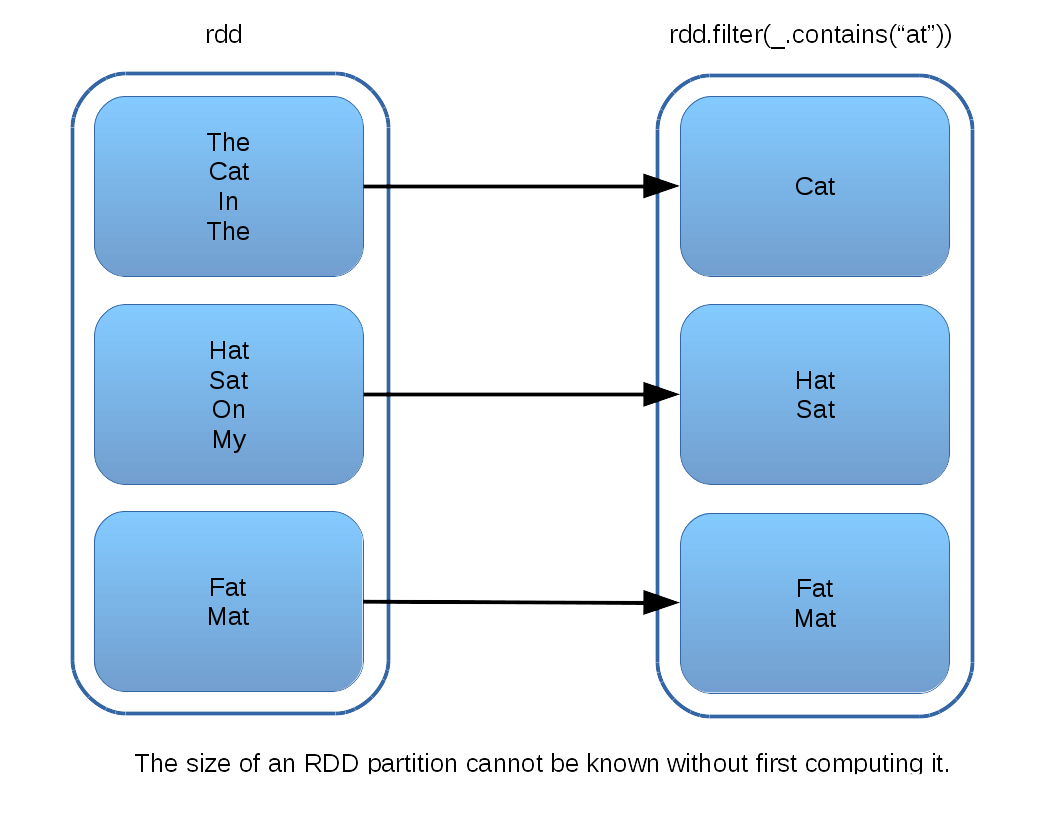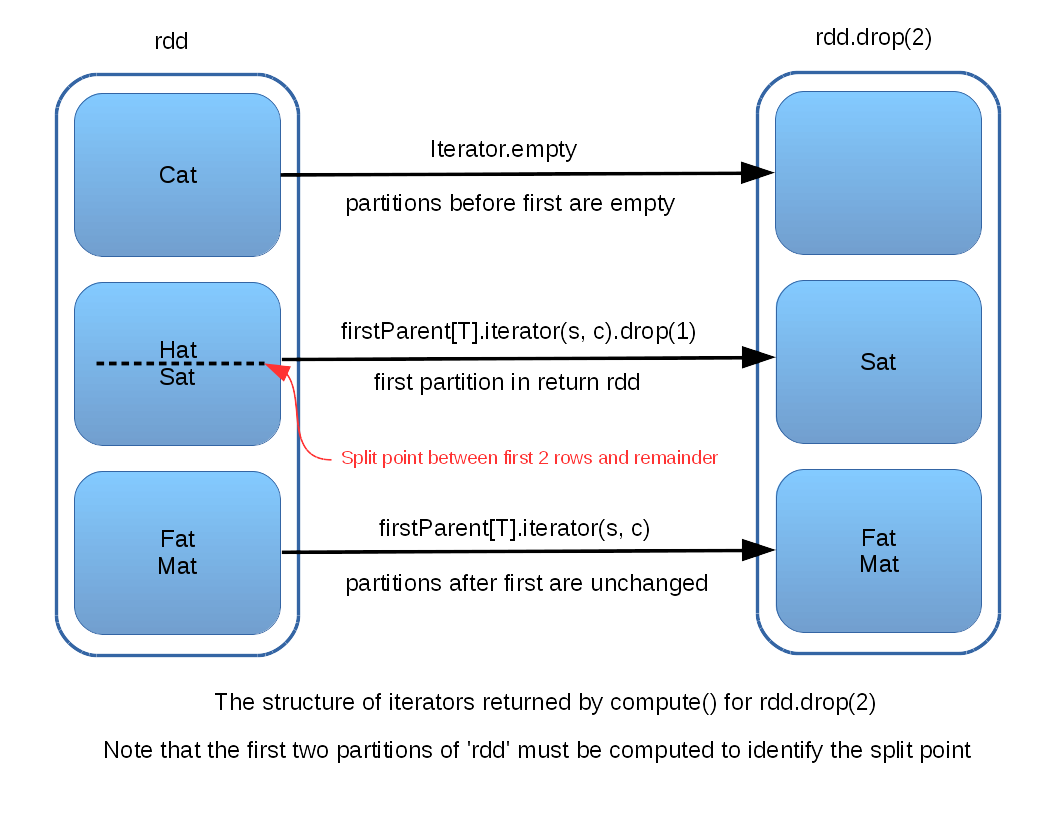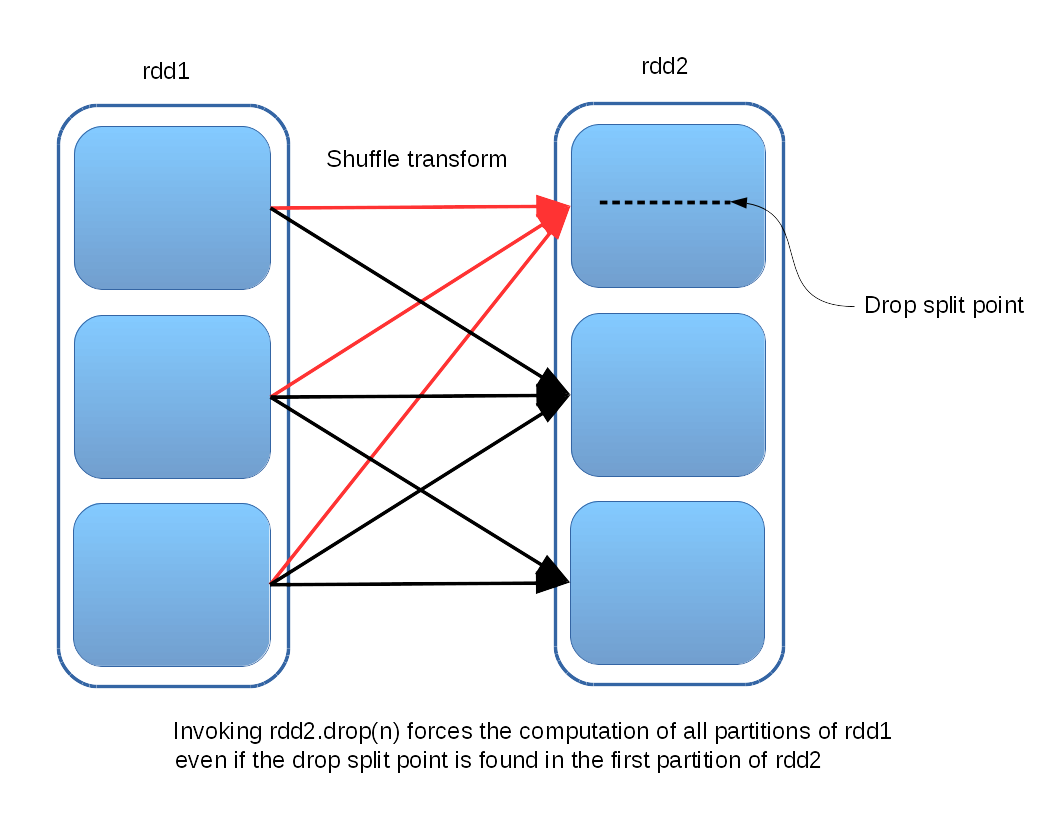In Scala, sequence data types support the drop method for skipping (aka “dropping”) the first elements of the sequence:

// drop the first element of a list
scala> List(1, 2, 3).drop(1)
res1: List[Int] = List(2, 3)


Spark RDDs also support various standard sequence methods, for example filter, as they are logically a sequence of row objects. One might suppose that drop could be a useful sequence method for RDDs, as it would support useful idioms like:

// Use drop (hypothetically) to skip the header of a text file:
val data = sparkContext.textFile("data.txt").drop(1)


Implementing drop for RDDs is possible, and in fact can be done with a small amount of code, however it comes at the price of an impact to the RDD lazy computing model.

To see why, recall that RDDs are composed of partitions, and so in order to drop the first (n) rows of an RDD, one must first identify the partition that contains the (n-1),(n) row boundary. In the resulting RDD, this partition will be the first one to contain any data. Identifying this “boundary” partition cannot have a closed-form solution, because partition sizes are not in general equal; the partition interface does not even support the concept of a count method. In order to obtain the size of a partition, one is forced to actually compute its contents. The diagram below illustrates one example of why this is so – the contents of the partitions in the filtered RDD on the right cannot be known without actually running the filter on the parent RDD:Given all this, the structure of a drop implementation is to compute the first partition, find its length, and see if it contains the requested (n-1),(n) boundary. If not, compute the next partition, and so on, until the boundary partition is identified. All prior partitions are ignored in the result. All subsequent partitions are passed on with no change. The boundary partition is passed through its own drop to eliminate rows up to (n).

The code implementing the concept described above can be viewed here: https://github.com/erikerlandson/spark/compare/erikerlandson:rdd_drop_blogpost_base…rdd_drop_blogpost

The following diagram illustrates the relation between input and output partitions in a call to drop:Arguably, this represents a potential subversion of the RDD lazy compute model, as it forces the computation of at least one (and possibly more) partitions. It behaves like a “partial action”, instead of a transform, but an action that returns another RDD.

In many cases, the impact of this might be relatively small. For example, dropping the first few rows in a text file is likely to only force computation of a single partition, and it is a partition that will eventually be computed anyway. Furthermore, such a use case is generally not inside a tight loop.

However, it is not hard to construct cases where computing even the first partition of one RDD recursively forces the computation of all the partitions in its parents, as in this example:Whether the benefits of supporting drop for RDDs outweigh the costs is an open question. It is likely to depend on whether or not the Spark community yields any compelling use cases for drop, and whether a transform that behaves like a “partial action” is considered an acceptable addition to the RDD formalism.

RDD support for drop has been proposed as issue SPARK-2315, with corresponding pull request 1254.